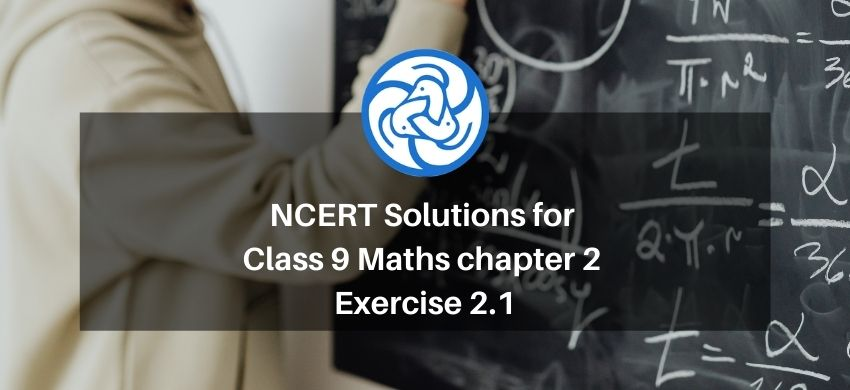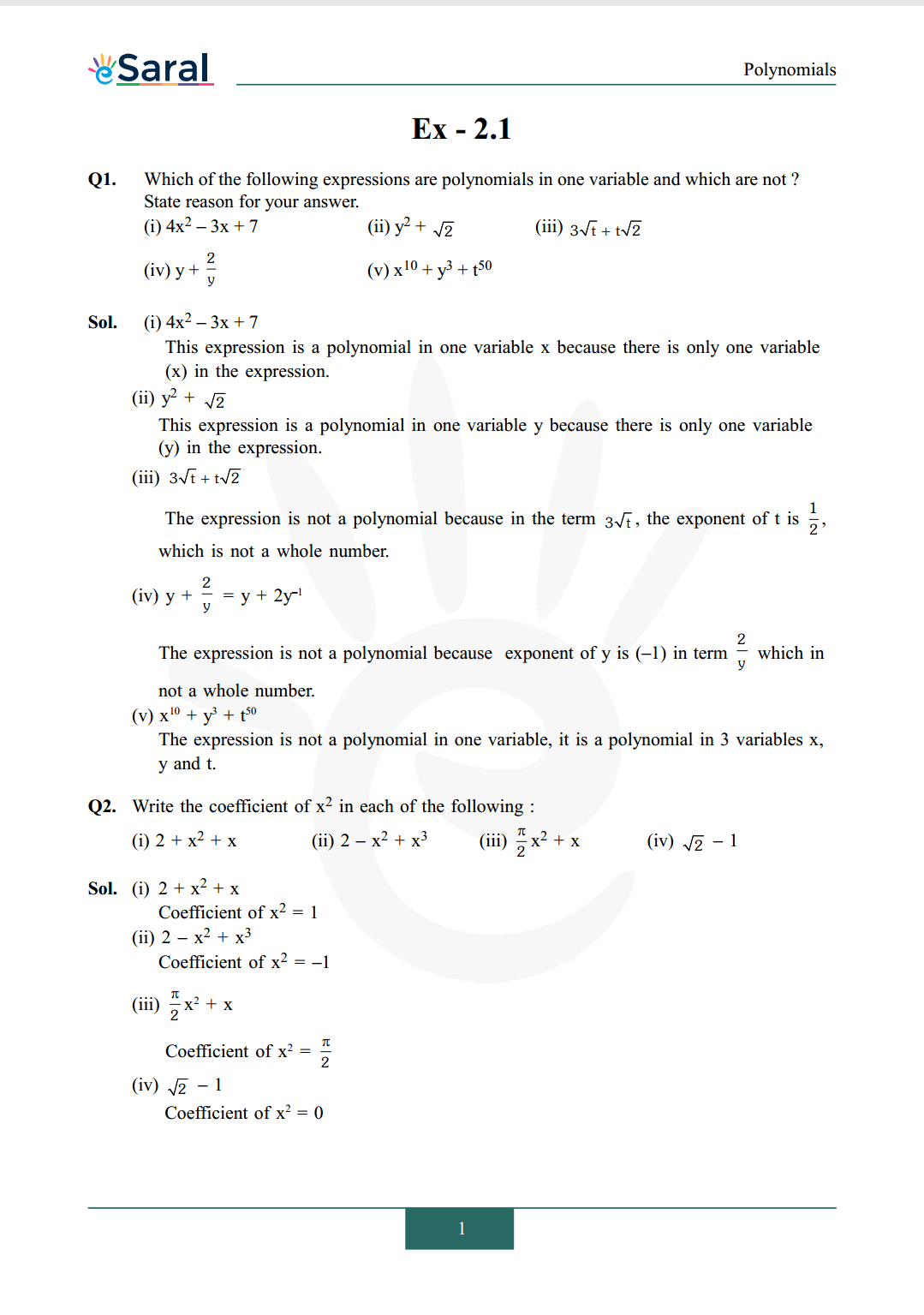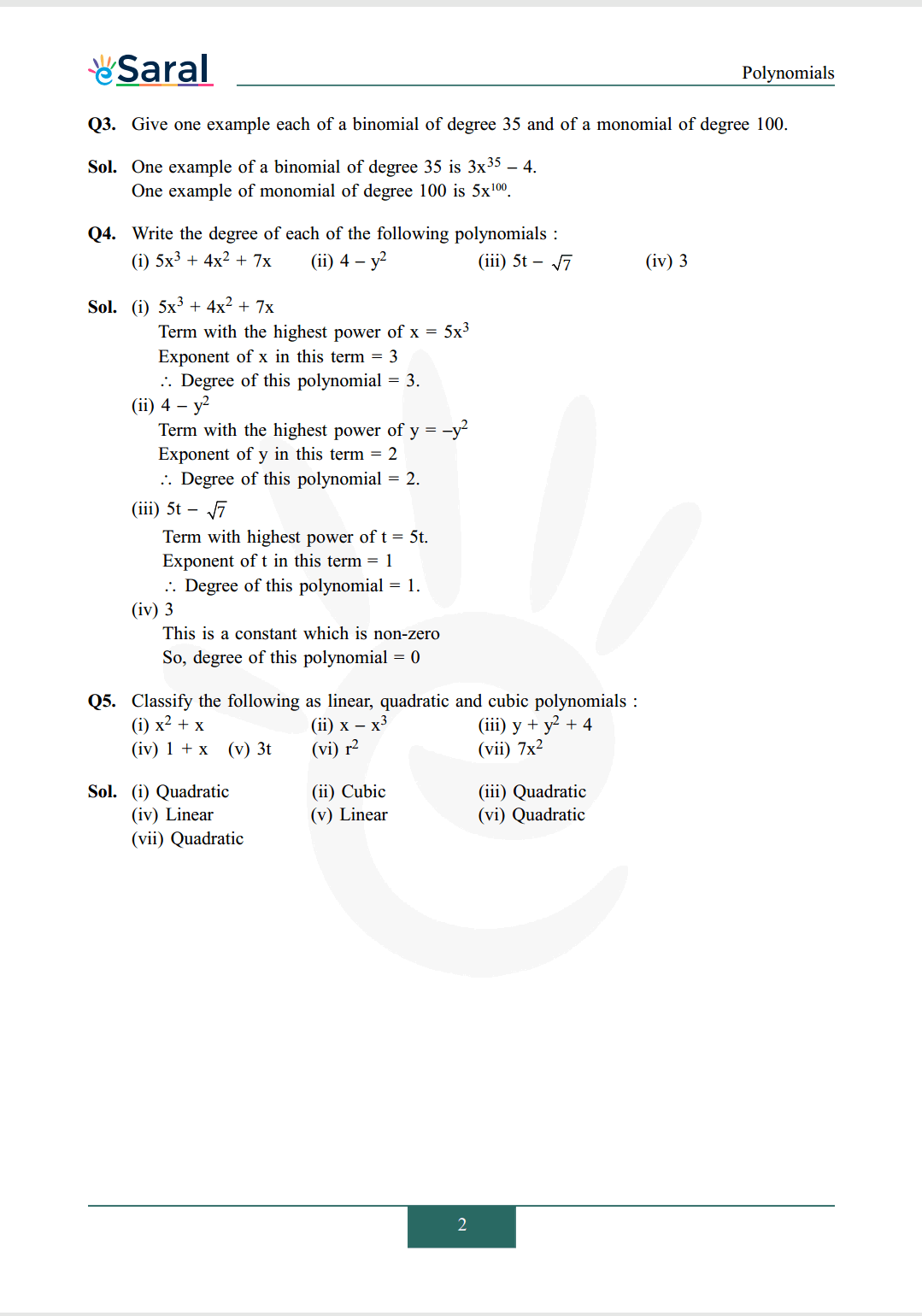Download the eSaral app and start learning from Kota's top IITians and doctors.

# NCERT Solutions for Class 9 Maths chapter 2 Exercise 2.1 - Polynomials - Free PDF DownloadHey, are you a class 9 Student and Looking for Ways to Download NCERT Solutions for Class 9 Maths chapter 2 Exercise 2.1? If Yes then you are at the right place.

Here we have listed Class 9 maths chapter 2 exercise 2.1 solutions  in PDF that is prepared by Kota’s top IITian’s Faculties by keeping Simplicity in mind.

If you want to score high in your class 9 Maths Exam then it is very important for you to have a good knowledge of all the important topics, so to learn and practice those topics you can use eSaral NCERT Solutions.

So, without wasting more time Let’s start.

### Download The PDF of NCERT Solutions for Class 9 Maths chapter 2 Exercise 2.1 "Polynomials"#### All Questions of Chapter 2 Exercise 2.1

Once you complete the chapter 2 then you can revise Ex. 2.1 by solving following questions

Q1. Which of the following expressions are polynomials in one variable and which are not ? State reason for your answer.
(i) $4 x^{2}-3 x+7$
(ii) $\mathrm{y}^{2}+\sqrt{2}$
(iii) $3 \sqrt{t}+t \sqrt{2}$
(iv) $y+\frac{2}{y}$
(v) $x^{10}+y^{3}+t^{50}$

Q2. Write the coefficient of $\mathrm{x}^{2}$ in each of the following :
(i) $2+x^{2}+x$
(ii) $2-x^{2}+x^{3}$
(iii) $\frac{\pi}{2} x^{2}+x$
(iv) $\sqrt{2}-1$

Q3. Give one example each of a binomial of degree 35 and of a monomial of degree 100.

Q4. Write the degree of each of the following polynomials :
(i) $5 x^{3}+4 x^{2}+7 x$
(ii) $4-y^{2}$
(iii) $5 \mathrm{t}-\sqrt{7}$
(iv) 3

Q5. Classify the following as linear, quadratic and cubic polynomials :
(i) $x^{2}+x$
(ii) $x-x^{3}$
(iii) $\mathrm{y}+\mathrm{y}^{2}+4$
(iv) $1+x$
(v) $3 \mathrm{t}$
(vi) $\mathrm{r}^{2}$
(vii) $7 \mathrm{x}^{2}$